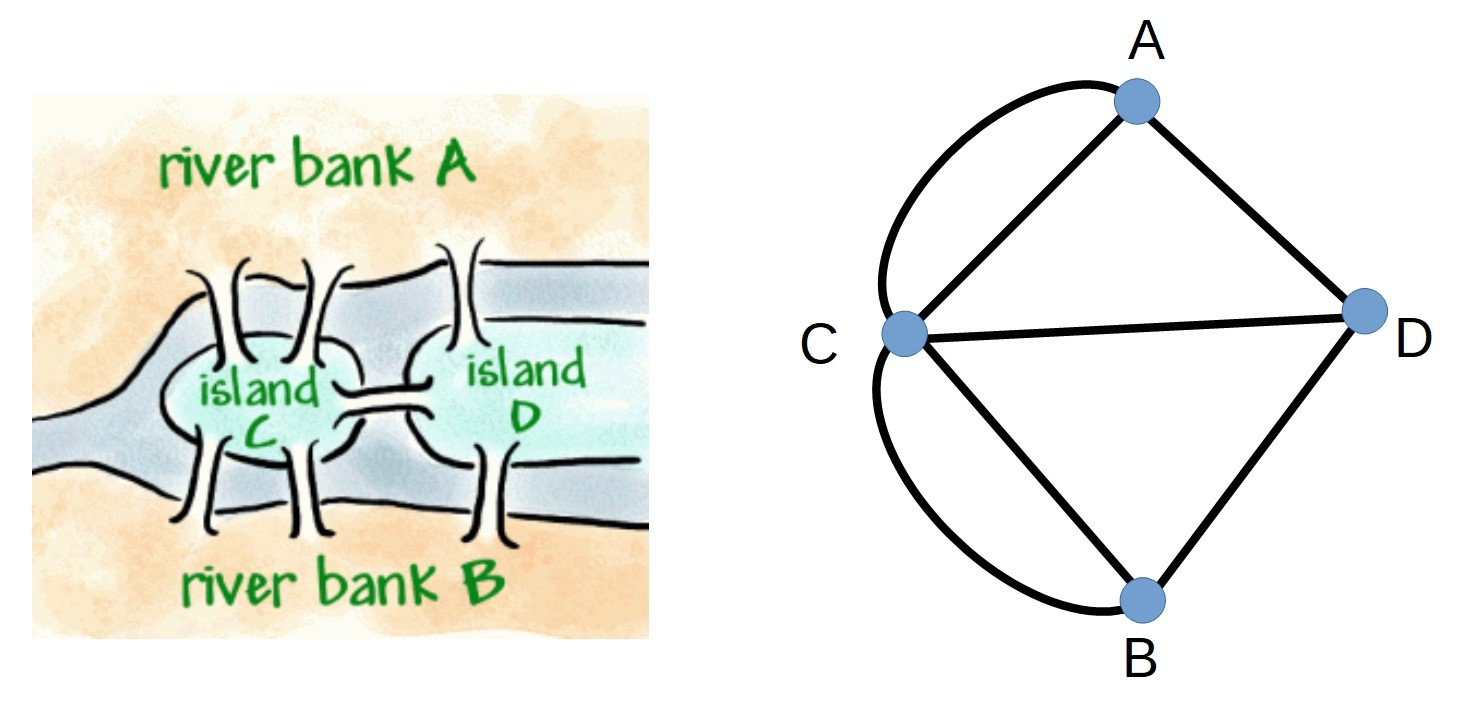#### You may also like### Calendar Capers

Choose any three by three square of dates on a calendar page...Make a set of numbers that use all the digits from 1 to 9, once and once only. Add them up. The result is divisible by 9. Add each of the digits in the new number. What is their sum? Now try some other possibilities for yourself!### Doodles

Draw a 'doodle' - a closed intersecting curve drawn without taking pencil from paper. What can you prove about the intersections?

# The Bridges of Konigsberg

##### Age 11 to 18Challenge Level

Konigsberg (now called Kaliningrad) is a town which lies on both sides of the Pregel River, and there are also parts of the town on two large islands that lie in the river. In the 18th century the river banks and islands were connected with seven bridges (as shown below).A popular problem of the time was whether or not it was possible to go for a walk around Konigsberg crossing each bridge exactly once.

Can you find a route around Konigsberg which crosses each bridge exactly once?

You will probably find, as the 18th Century inhabitants of Konigsberg did, that this is not possible!

Here are two more questions:

• Can you remove a bridge and find a walk that crosses each remaining bridge exactly once?
• Can you add a bridge and find a walk that crosses all the bridges exactly once?

To simplify the problem, we can represent Konigsberg by a network of vertices and edges, where each vertex represents one of the land masses (the river banks and islands) and each edge represents a bridge.Try adding another edge (bridge) between vertices A and D. Draw out this new network.

• Can you now find a route which uses each edge exactly once?
• Are there any restrictions on which vertex you can start from?
• Can you add one (or more) edges to the original network so that there is a walk which crosses every bridge exactly once, and where you finish at the same vertex where you started?

To find out more about when it is possible to travel along every edge of a network exactly once, try the problem "Can You Traverse It?".

You can read more about the Bridges of Konigsberg in this Maths In a Minute Plus article.
To find out how this problem developed into graph theory go to From Bridges to Networks Plus article.